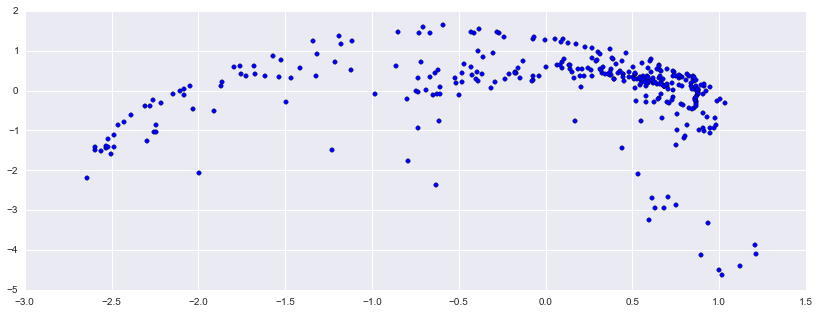In :
import numpy, scipy, matplotlib.pyplot as plt, sklearn, urllib, stanford_mir, IPython.display
%matplotlib inline
plt.rcParams['figure.figsize'] = (14, 5)


# Principal Component Analysis¶

In :
filename = '125_bounce.wav'

In :
url = 'http://audio.musicinformationretrieval.com/'
urllib.urlretrieve(url + filename, filename=filename)

Out:
('125_bounce.wav', <httplib.HTTPMessage instance at 0x109afb248>)

In :
x, fs = librosa.load(filename)


Listen to the signal:

In :
IPython.display.Audio(x, rate=fs)

Out:

Compute some features:

In :
X = librosa.feature.mfcc(x, sr=fs)

In :
print X.shape

(20, 331)


Scale the features to have zero mean and unit variance:

In :
X = sklearn.preprocessing.scale(X)

In :
X.mean()

Out:
-4.2933095816320253e-18

Create a PCA model object.

In :
model = sklearn.decomposition.PCA(n_components=2, whiten=True)


Apply PCA to the scaled features:

In :
model.fit(X.T)

Out:
PCA(copy=True, n_components=2, whiten=True)
In :
Y = model.transform(X.T)

In :
print Y.shape

(331, 2)


Let's see how many principal components were returned:

In :
model.components_.shape

Out:
(2, 20)

Plot the two top principal components for each data point:

In :
plt.scatter(Y[:,0], Y[:,1])

Out:
<matplotlib.collections.PathCollection at 0x10aebbc10>